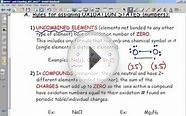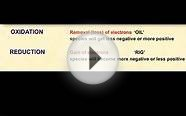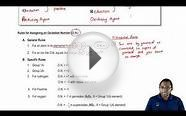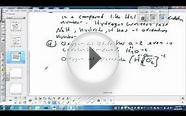# Rules of oxidation

Oxidation numbers are bookkeeping numbers. They allow chemists to do things such as balance redox (reduction/oxidation) equations. Oxidation numbers are positive or negative numbers, but don’t confuse them with positive or negative charges on ions or valences.

Oxidation numbers are assigned to elements using these rules:

• Rule 1: The oxidation number of an element in its free (uncombined) state is zero — for example, Al(s) or Zn(s). This is also true for elements found in nature as diatomic (two-atom) elements

and for sulfur, found as:

• Rule 2: The oxidation number of a monatomic (one-atom) ion is the same as the charge on the ion, for example:
• Rule 3: The sum of all oxidation numbers in a neutral compound is zero. The sum of all oxidation numbers in a polyatomic (many-atom) ion is equal to the charge on the ion. This rule often allows chemists to calculate the oxidation number of an atom that may have multiple oxidation states, if the other atoms in the ion have known oxidation numbers.
• Rule 4: The oxidation number of an alkali metal (IA family) in a compound is +1; the oxidation number of an alkaline earth metal (IIA family) in a compound is +2.
• Rule 5: The oxidation number of oxygen in a compound is usually –2. If, however, the oxygen is in a class of compounds called peroxides (for example, hydrogen peroxide), then the oxygen has an oxidation number of –1. If the oxygen is bonded to fluorine, the number is +1.
• Rule 6: The oxidation state of hydrogen in a compound is usually +1. If the hydrogen is part of a binary metal hydride (compound of hydrogen and some metal), then the oxidation state of hydrogen is –1.
• Rule 7: The oxidation number of fluorine is always –1. Chlorine, bromine, and iodine usually have an oxidation number of –1, unless they’re in combination with an oxygen or fluorine.

These rules give you another way to define oxidation and reduction — in terms of oxidation numbers. For example, consider this reaction, which shows oxidation by the loss of electrons:

Notice that the zinc metal (the reactant) has an oxidation number of zero (rule 1), and the zinc cation (the product) has an oxidation number of +2 (rule 2). In general, you can say that a substance is oxidized when there’s an increase in its oxidation number.

###### You might also likeOxidation State and Rules for Assigning Oxidation StateBonding: Oxidation Numbers (Part 1: Rules)Redox 1- oxidation, reduction and oxidation state rulesRules for Oxidation Numbersrules of oxidation numbersOxidation rules
Related Posts